# January 2012 Regents Exam http www nysedregents orgchemistry

• Slides: 51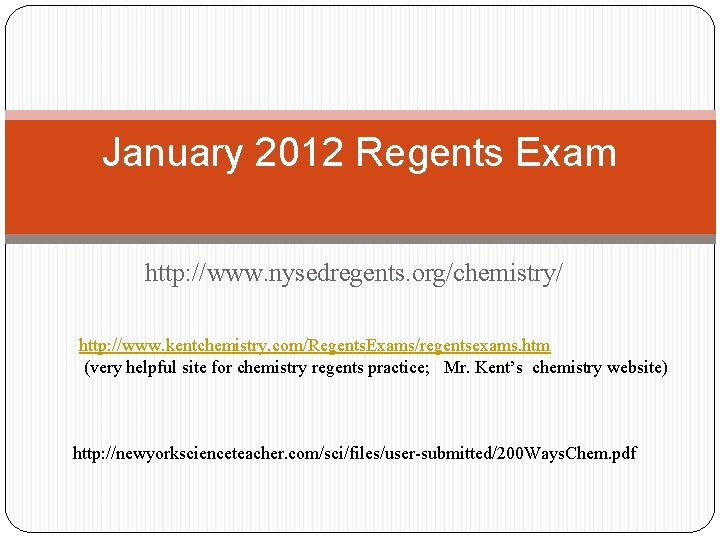January 2012 Regents Exam http: //www. nysedregents. org/chemistry/ http: //www. kentchemistry. com/Regents. Exams/regentsexams. htm (very helpful site for chemistry regents practice; Mr. Kent’s chemistry website) http: //newyorkscienceteacher. com/sci/files/user-submitted/200 Ways. Chem. pdfJanuary 2012 Regents Exam Part A Questions http: //www. nysedregents. org/chemistry/ http: //www. kentchemistry. com/Regents. Exams/regentsexams. htm (very helpful site for chemistry regents practice; Mr. Kent’s chemistry website) http: //newyorkscienceteacher. com/sci/files/user-submitted/200 Ways. Chem. pdfJanuary 2012 Questions Part A Q 1 -6 are based on Atom/Atomic Theory (items 1 - 16 of the 200 Ways to Pass …. ) What is the number of electrons in a completely filled second shell of an atom? (1) 32 (2) 2 (3) 18 (4) 8 What is the number of electrons in an atom that has 3 protons and 4 neutrons? (1) 1 (2) 7 (3) 3 (4) 4 As a result of the gold foil experiment, it was concluded that an atom (1) contains protons, neutrons, and electrons (2) contains a small, dense nucleus (3) has positrons and orbitals and (4) is a hard, indivisible sphere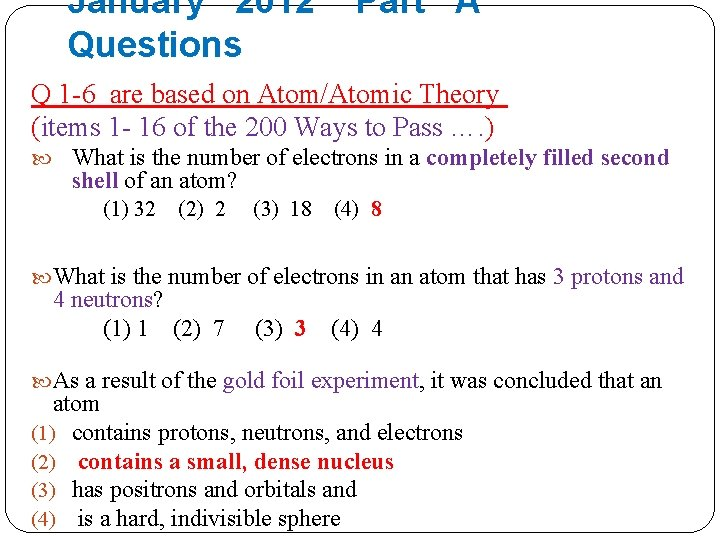January 2012 Questions Part A Q 1 -6 are based on Atom/Atomic Theory (items 1 - 16 of the 200 Ways to Pass …. ) What is the number of electrons in a completely filled second shell of an atom? (1) 32 (2) 2 (3) 18 (4) 8 What is the number of electrons in an atom that has 3 protons and 4 neutrons? (1) 1 (2) 7 (3) 3 (4) 4 As a result of the gold foil experiment, it was concluded that an atom (1) contains protons, neutrons, and electrons (2) contains a small, dense nucleus (3) has positrons and orbitals and (4) is a hard, indivisible sphereQ 1 -6 are based on Atom/Atomic Theory Which statement describes the distribution of charge in an atom? (l) A neutral nucleus is surrounded by one or more negatively charged electrons. (2) A neutral nucleus is surrounded by one or more positively charged electrons. (3) A positively charged nucleus is surrounded by one or more negatively charged electrons. (4) A positively charged nucleus is surrounded by one or more positively charged electrons. Which atom in the ground state has an outermost electron with the most energy? (1) Cs (3) Li (2) K (4) Na Which particle has the least mass? (1) alpha particle (3) neutron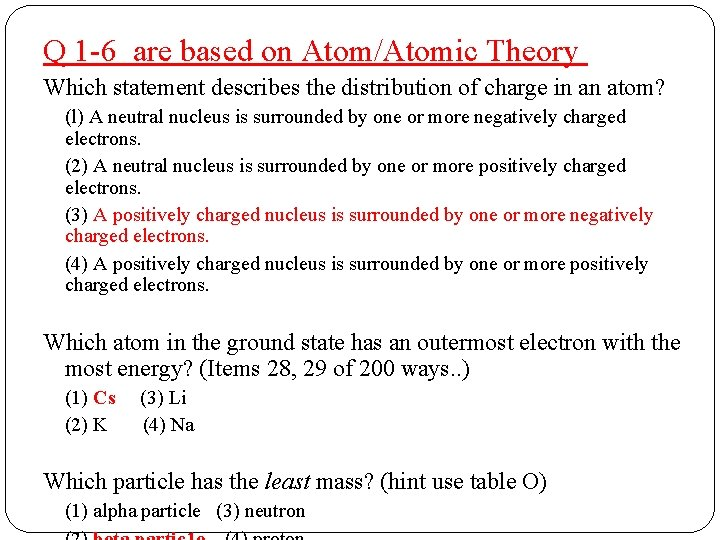Q 1 -6 are based on Atom/Atomic Theory Which statement describes the distribution of charge in an atom? (l) A neutral nucleus is surrounded by one or more negatively charged electrons. (2) A neutral nucleus is surrounded by one or more positively charged electrons. (3) A positively charged nucleus is surrounded by one or more negatively charged electrons. (4) A positively charged nucleus is surrounded by one or more positively charged electrons. Which atom in the ground state has an outermost electron with the most energy? (Items 28, 29 of 200 ways. . ) (1) Cs (3) Li (2) K (4) Na Which particle has the least mass? (hint use table O) (1) alpha particle (3) neutron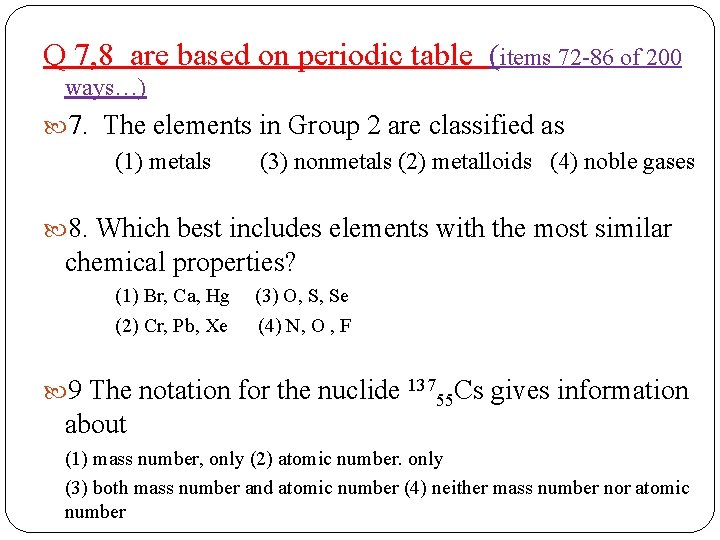Q 7, 8 are based on periodic table (items 72 -86 of 200 ways…) 7. The elements in Group 2 are classified as (1) metals (3) nonmetals (2) metalloids (4) noble gases 8. Which best includes elements with the most similar chemical properties? (1) Br, Ca, Hg (3) O, S, Se (2) Cr, Pb, Xe (4) N, O , F 9 The notation for the nuclide 13755 Cs gives information about (1) mass number, only (2) atomic number. only (3) both mass number and atomic number (4) neither mass number nor atomic numberQ 9 based on item 27 of 200 ways to pass…. . ) 7. The elements in Group 2 are classified as (1) metals (3) nonmetals (2) metalloids (4) noble gases 8. Which best includes elements with the most similar chemical properties? (Hint look for elements in same group) (1) Br, Ca, Hg (3) O, S, Se (2) Cr, Pb, Xe (4) N, O , F 9. The notation for the nuclide 13755 Cs gives information about (1) mass number, only (2) atomic number. only (3) both mass number and atomic number (4) neither mass number nor atomic number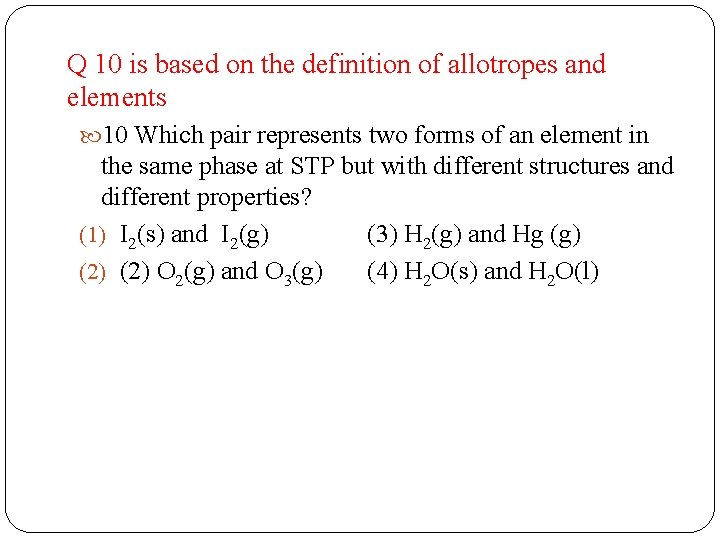Q 10 is based on the definition of allotropes and elements 10 Which pair represents two forms of an element in the same phase at STP but with different structures and different properties? (1) I 2(s) and I 2(g) (3) H 2(g) and Hg (g) (2) O 2(g) and O 3(g) (4) H 2 O(s) and H 2 O(l)Q 10 is based on the definition of allotropes and elements 10 Which pair represents two forms of an element in the same phase at STP but with different structures and different properties? (1) I 2(s) and I 2(g) (3) H 2(g) and Hg (g) (2) O 2(g) and O 3(g) (4) H 2 O(s) and H 2 O(l)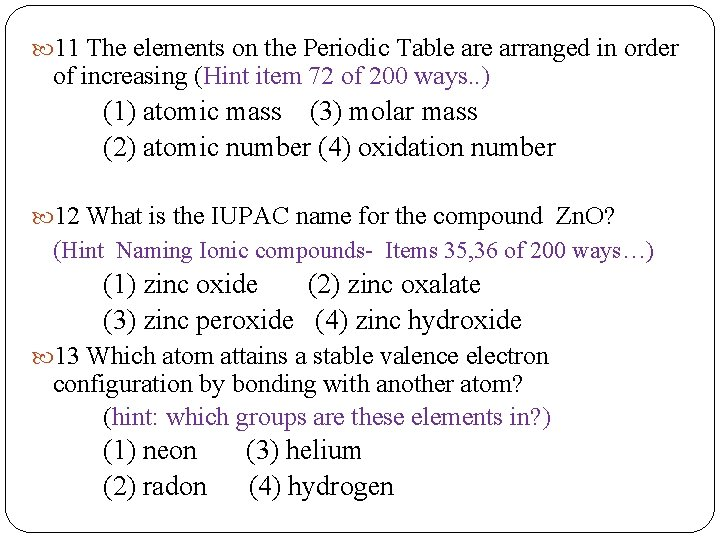11 The elements on the Periodic Table arranged in order of increasing (Hint item 72 of 200 ways. . ) (1) atomic mass (3) molar mass (2) atomic number (4) oxidation number 12 What is the IUPAC name for the compound Zn. O? (Hint Naming Ionic compounds- Items 35, 36 of 200 ways…) (1) zinc oxide (2) zinc oxalate (3) zinc peroxide (4) zinc hydroxide 13 Which atom attains a stable valence electron configuration by bonding with another atom? (hint: which groups are these elements in? ) (1) neon (3) helium (2) radon (4) hydrogen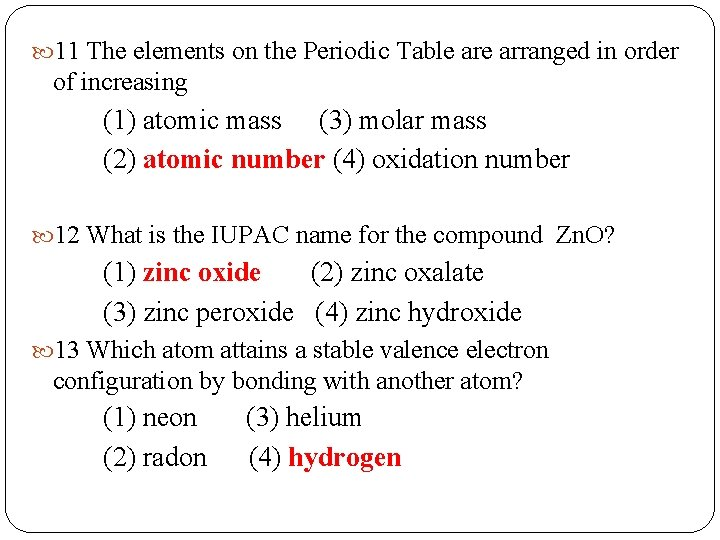11 The elements on the Periodic Table arranged in order of increasing (1) atomic mass (3) molar mass (2) atomic number (4) oxidation number 12 What is the IUPAC name for the compound Zn. O? (1) zinc oxide (2) zinc oxalate (3) zinc peroxide (4) zinc hydroxide 13 Which atom attains a stable valence electron configuration by bonding with another atom? (1) neon (3) helium (2) radon (4) hydrogen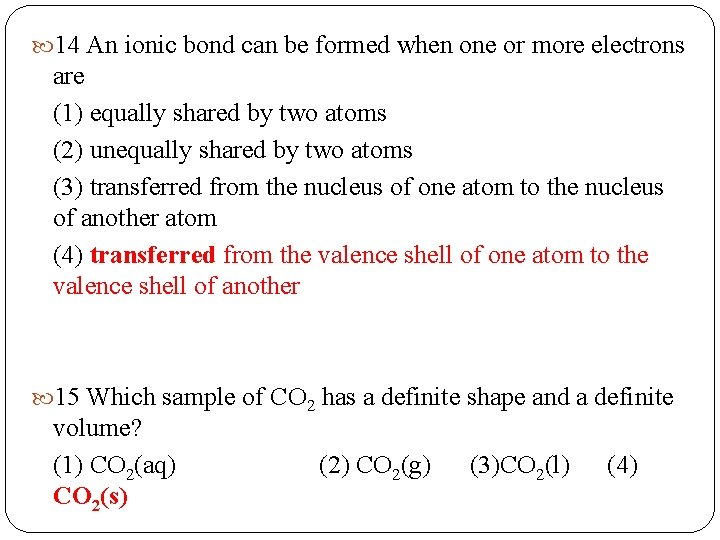14 An ionic bond can be formed when one or more electrons are (1) equally shared by two atoms (2) unequally shared by two atoms (3) transferred from the nucleus of one atom to the nucleus of another atom (4) transferred from the valence shell of one atom to the valence shell of another 15 Which sample of CO 2 has a definite shape and a definite volume? (1) CO 2(aq) CO 2(s) (2) CO 2(g) (3)CO 2(l) (4)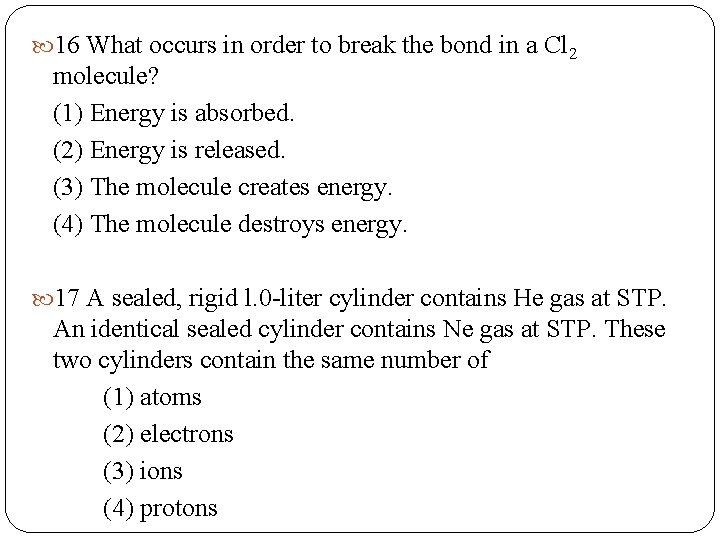16 What occurs in order to break the bond in a Cl 2 molecule? (1) Energy is absorbed. (2) Energy is released. (3) The molecule creates energy. (4) The molecule destroys energy. 17 A sealed, rigid l. 0 -liter cylinder contains He gas at STP. An identical sealed cylinder contains Ne gas at STP. These two cylinders contain the same number of (1) atoms (2) electrons (3) ions (4) protons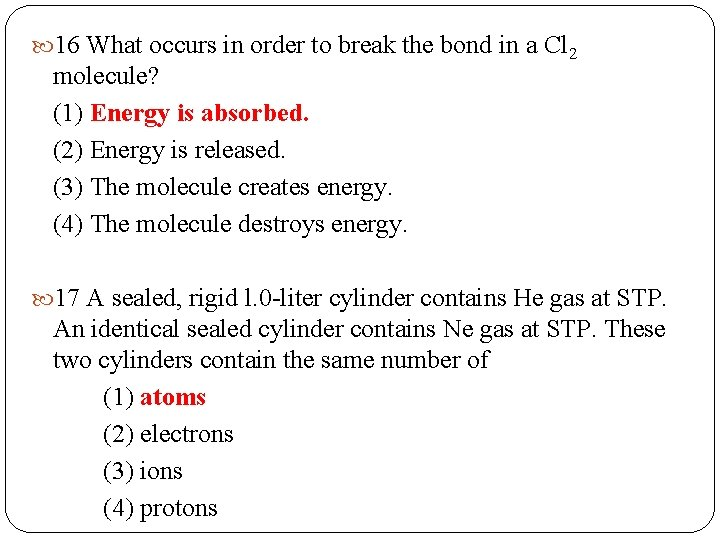16 What occurs in order to break the bond in a Cl 2 molecule? (1) Energy is absorbed. (2) Energy is released. (3) The molecule creates energy. (4) The molecule destroys energy. 17 A sealed, rigid l. 0 -liter cylinder contains He gas at STP. An identical sealed cylinder contains Ne gas at STP. These two cylinders contain the same number of (1) atoms (2) electrons (3) ions (4) protons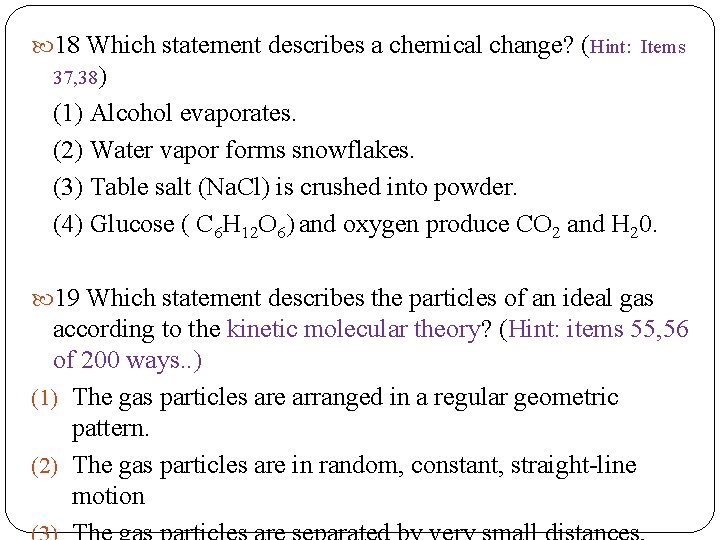18 Which statement describes a chemical change? (Hint: Items 37, 38) (1) Alcohol evaporates. (2) Water vapor forms snowflakes. (3) Table salt (Na. Cl) is crushed into powder. (4) Glucose ( C 6 H 12 O 6) and oxygen produce CO 2 and H 20. 19 Which statement describes the particles of an ideal gas according to the kinetic molecular theory? (Hint: items 55, 56 of 200 ways. . ) (1) The gas particles are arranged in a regular geometric pattern. (2) The gas particles are in random, constant, straight-line motion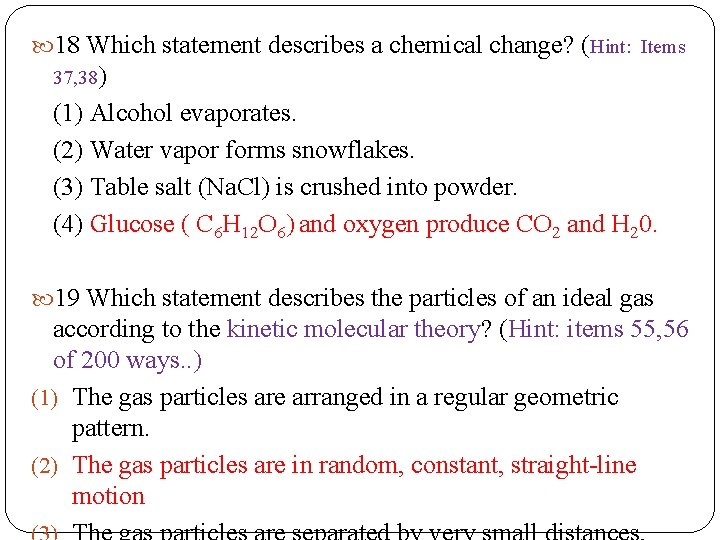18 Which statement describes a chemical change? (Hint: Items 37, 38) (1) Alcohol evaporates. (2) Water vapor forms snowflakes. (3) Table salt (Na. Cl) is crushed into powder. (4) Glucose ( C 6 H 12 O 6) and oxygen produce CO 2 and H 20. 19 Which statement describes the particles of an ideal gas according to the kinetic molecular theory? (Hint: items 55, 56 of 200 ways. . ) (1) The gas particles are arranged in a regular geometric pattern. (2) The gas particles are in random, constant, straight-line motion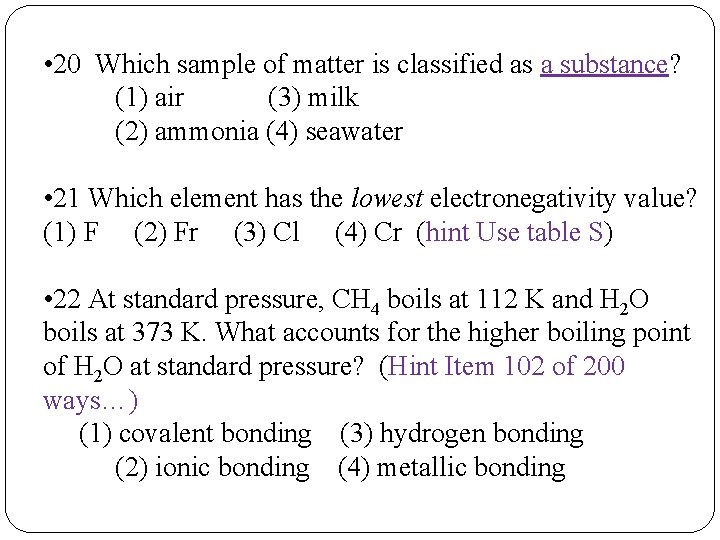• 20 Which sample of matter is classified as a substance? (1) air (3) milk (2) ammonia (4) seawater • 21 Which element has the lowest electronegativity value? (1) F (2) Fr (3) Cl (4) Cr (hint Use table S) • 22 At standard pressure, CH 4 boils at 112 K and H 2 O boils at 373 K. What accounts for the higher boiling point of H 2 O at standard pressure? (Hint Item 102 of 200 ways…) (1) covalent bonding (3) hydrogen bonding (2) ionic bonding (4) metallic bonding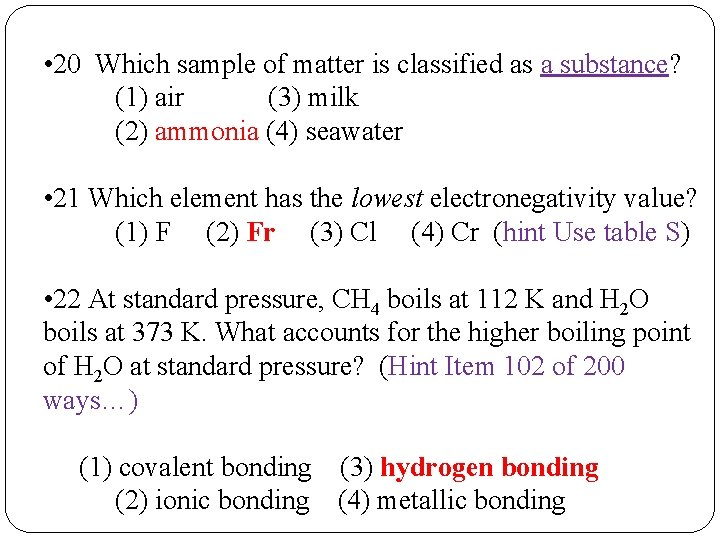• 20 Which sample of matter is classified as a substance? (1) air (3) milk (2) ammonia (4) seawater • 21 Which element has the lowest electronegativity value? (1) F (2) Fr (3) Cl (4) Cr (hint Use table S) • 22 At standard pressure, CH 4 boils at 112 K and H 2 O boils at 373 K. What accounts for the higher boiling point of H 2 O at standard pressure? (Hint Item 102 of 200 ways…) (1) covalent bonding (3) hydrogen bonding (2) ionic bonding (4) metallic bondingQuestion 23 based on separation of mixtures; Q 24 based on Enthalpy and Entropy (Items 130, 131 ) FACTS TO KNOW 23 A mixture of sand table salt can be separated by filtration because the substances in the mixture differ in ( 1) boiling point (2) density at STP (3) freezing point (4) solubility in water 24 Systems in nature toward tend to undergo changes (1) lower energy and lower entropy (2) lower energy and higher entropy (3) higher energy and lower entropy (4) higher energy and higher entropyQuestion 23 based on separation of mixtures; Q 24 based on Enthalpy and Entropy (Items 130, 131 ) FACTS TO KNOW 23 A mixture of sand table salt can be separated by filtration because the substances in the mixture differ in ( 1) boiling point (2) density at STP (3) freezing point (4) solubility in water 24 Systems in nature toward tend to undergo changes (1) lower energy and lower entropy (2) lower energy and higher entropy (3) higher energy and lower entropy (4) higher energy and higher entropy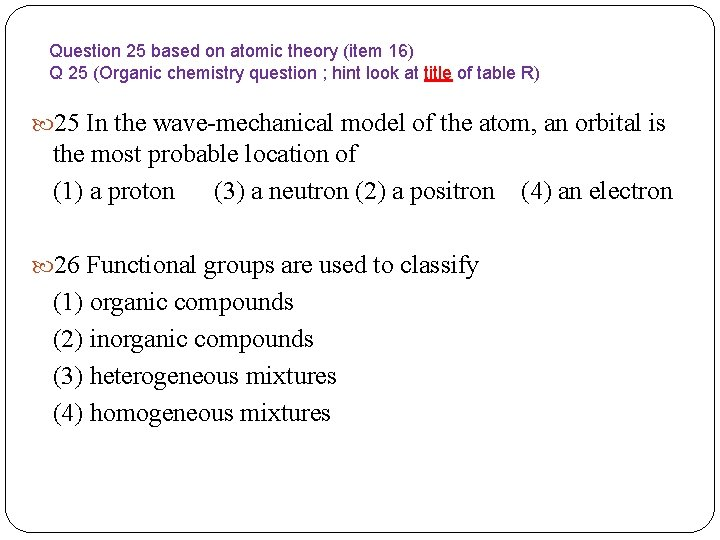Question 25 based on atomic theory (item 16) Q 25 (Organic chemistry question ; hint look at title of table R) 25 In the wave-mechanical model of the atom, an orbital is the most probable location of (1) a proton (3) a neutron (2) a positron (4) an electron 26 Functional groups are used to classify (1) organic compounds (2) inorganic compounds (3) heterogeneous mixtures (4) homogeneous mixtures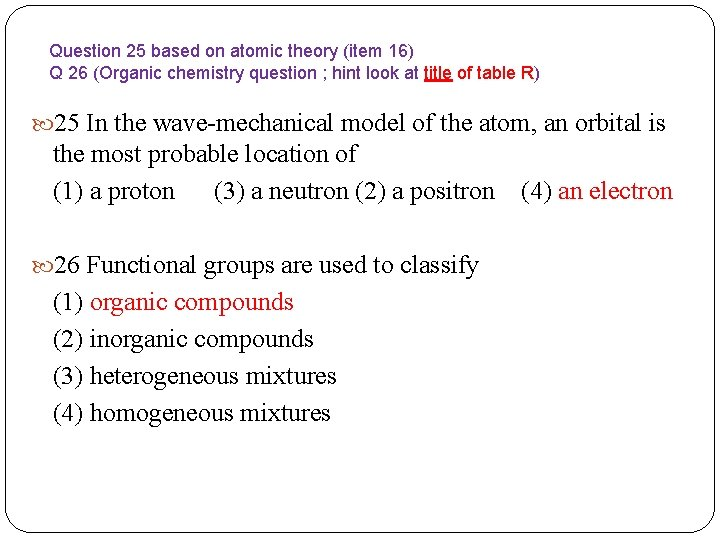Question 25 based on atomic theory (item 16) Q 26 (Organic chemistry question ; hint look at title of table R) 25 In the wave-mechanical model of the atom, an orbital is the most probable location of (1) a proton (3) a neutron (2) a positron (4) an electron 26 Functional groups are used to classify (1) organic compounds (2) inorganic compounds (3) heterogeneous mixtures (4) homogeneous mixtures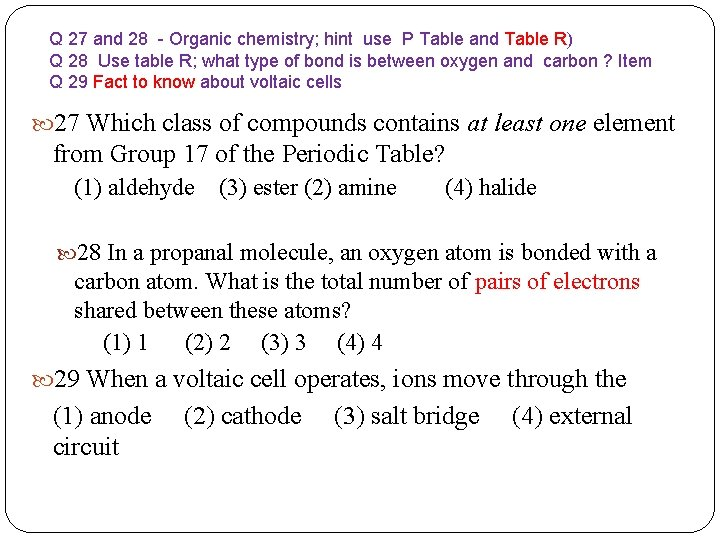Q 27 and 28 - Organic chemistry; hint use P Table and Table R) Q 28 Use table R; what type of bond is between oxygen and carbon ? Item Q 29 Fact to know about voltaic cells 27 Which class of compounds contains at least one element from Group 17 of the Periodic Table? (1) aldehyde (3) ester (2) amine (4) halide 28 In a propanal molecule, an oxygen atom is bonded with a carbon atom. What is the total number of pairs of electrons shared between these atoms? (1) 1 (2) 2 (3) 3 (4) 4 29 When a voltaic cell operates, ions move through the (1) anode (2) cathode (3) salt bridge (4) external circuit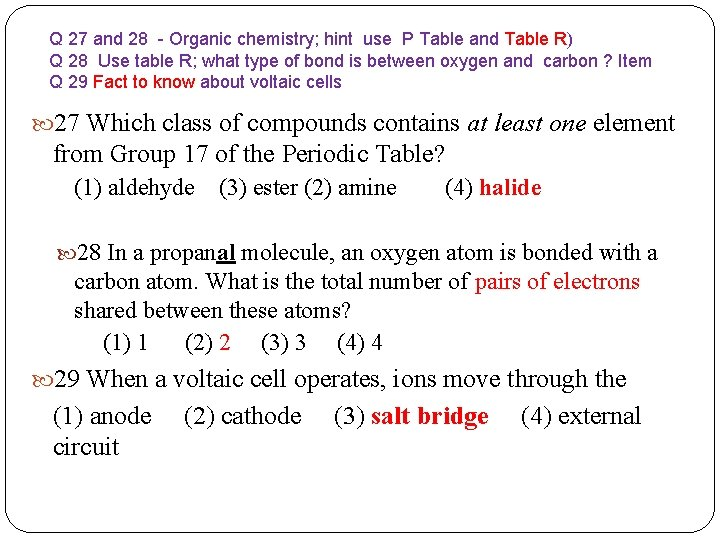Q 27 and 28 - Organic chemistry; hint use P Table and Table R) Q 28 Use table R; what type of bond is between oxygen and carbon ? Item Q 29 Fact to know about voltaic cells 27 Which class of compounds contains at least one element from Group 17 of the Periodic Table? (1) aldehyde (3) ester (2) amine (4) halide 28 In a propanal molecule, an oxygen atom is bonded with a carbon atom. What is the total number of pairs of electrons shared between these atoms? (1) 1 (2) 2 (3) 3 (4) 4 29 When a voltaic cell operates, ions move through the (1) anode (2) cathode (3) salt bridge (4) external circuit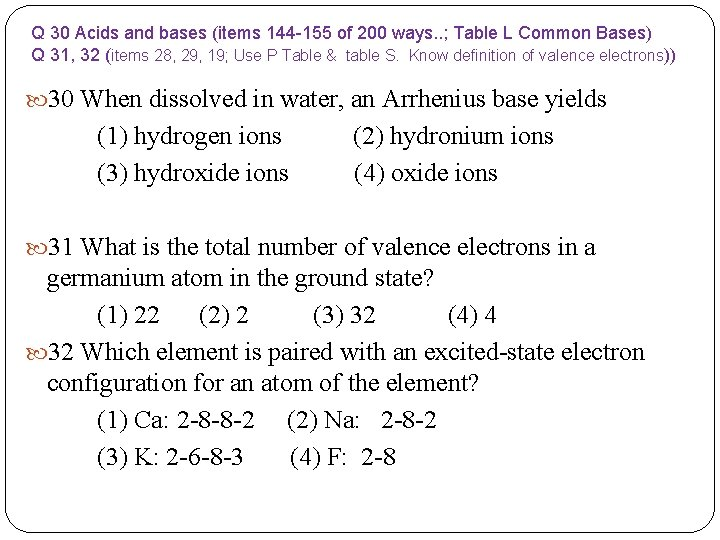Q 30 Acids and bases (items 144 -155 of 200 ways. . ; Table L Common Bases) Q 31, 32 (items 28, 29, 19; Use P Table & table S. Know definition of valence electrons)) 30 When dissolved in water, an Arrhenius base yields (1) hydrogen ions (2) hydronium ions (3) hydroxide ions (4) oxide ions 31 What is the total number of valence electrons in a germanium atom in the ground state? (1) 22 (2) 2 (3) 32 (4) 4 32 Which element is paired with an excited-state electron configuration for an atom of the element? (1) Ca: 2 -8 -8 -2 (2) Na: 2 -8 -2 (3) K: 2 -6 -8 -3 (4) F: 2 -8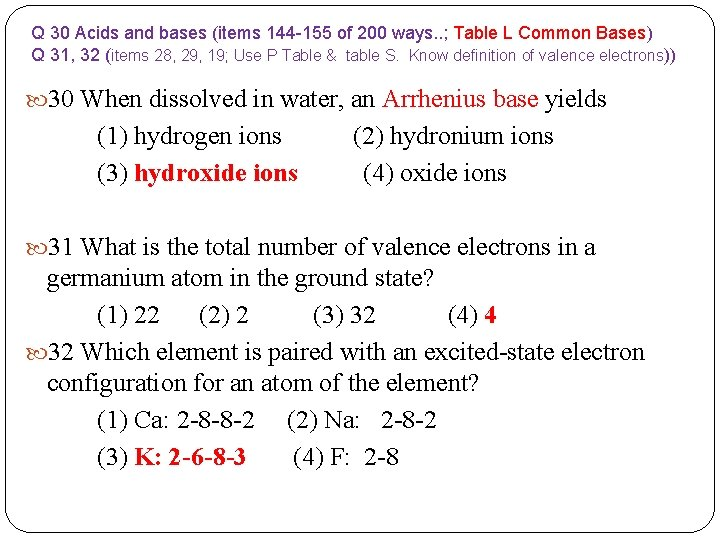Q 30 Acids and bases (items 144 -155 of 200 ways. . ; Table L Common Bases) Q 31, 32 (items 28, 29, 19; Use P Table & table S. Know definition of valence electrons)) 30 When dissolved in water, an Arrhenius base yields (1) hydrogen ions (2) hydronium ions (3) hydroxide ions (4) oxide ions 31 What is the total number of valence electrons in a germanium atom in the ground state? (1) 22 (2) 2 (3) 32 (4) 4 32 Which element is paired with an excited-state electron configuration for an atom of the element? (1) Ca: 2 -8 -8 -2 (2) Na: 2 -8 -2 (3) K: 2 -6 -8 -3 (4) F: 2 -8Q 33 based on types of reactions (TO KNOW- items 43 -46) 33 Given the balanced equations representing two chemical reactions: Cl 2 + 2 Na. Br ---> 2 Na. Cl + Br 2 2 Na. Cl---> 2 Na + Cl 2 Which types of chemical reactions are represented by these equations? (1) single replacement and decomposition (2) single replacement and double replacement (3) synthesis and decomposition (4) synthesis and double replacementQ 33 based on types of reactions (TO KNOW- items 43 -46) 33 Given the balanced equations representing two chemical reactions: Cl 2 + 2 Na. Br ---> 2 Na. Cl + Br 2 2 Na. Cl---> 2 Na + Cl 2 Which types of chemical reactions are represented by these equations? (1) single replacement and decomposition (2) single replacement and double replacement (3) synthesis and decomposition (4) synthesis and double replacement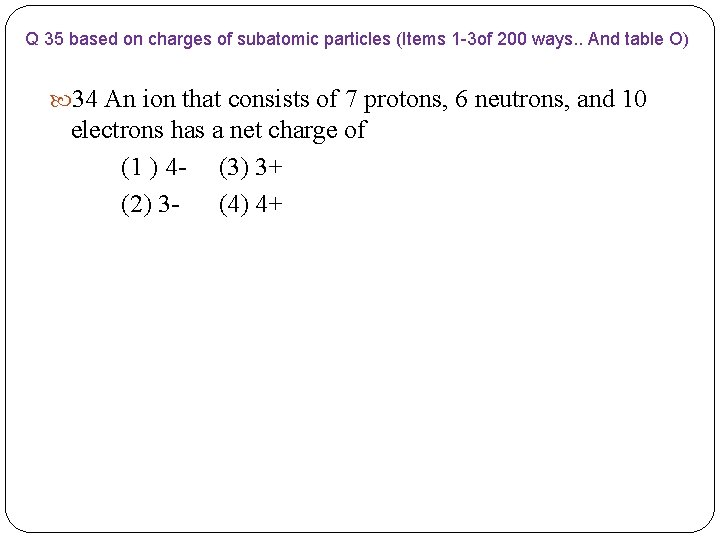Q 35 based on charges of subatomic particles (Items 1 -3 of 200 ways. . And table O) 34 An ion that consists of 7 protons, 6 neutrons, and 10 electrons has a net charge of (1 ) 4 - (3) 3+ (2) 3 - (4) 4+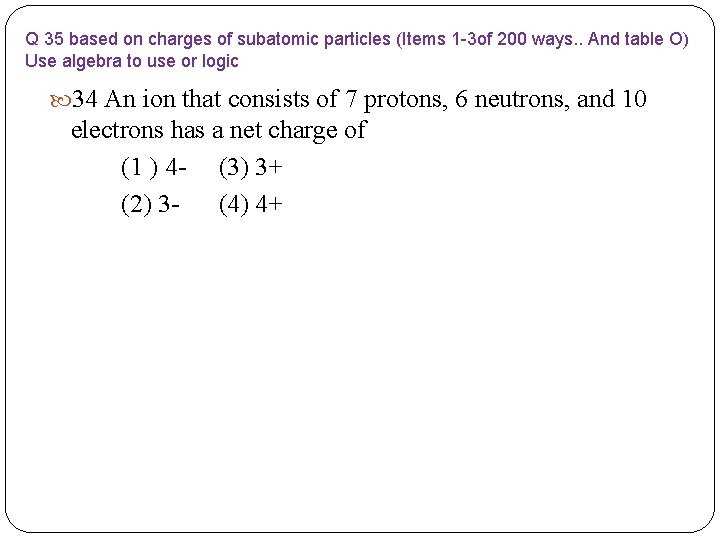Q 35 based on charges of subatomic particles (Items 1 -3 of 200 ways. . And table O) Use algebra to use or logic 34 An ion that consists of 7 protons, 6 neutrons, and 10 electrons has a net charge of (1 ) 4 - (3) 3+ (2) 3 - (4) 4+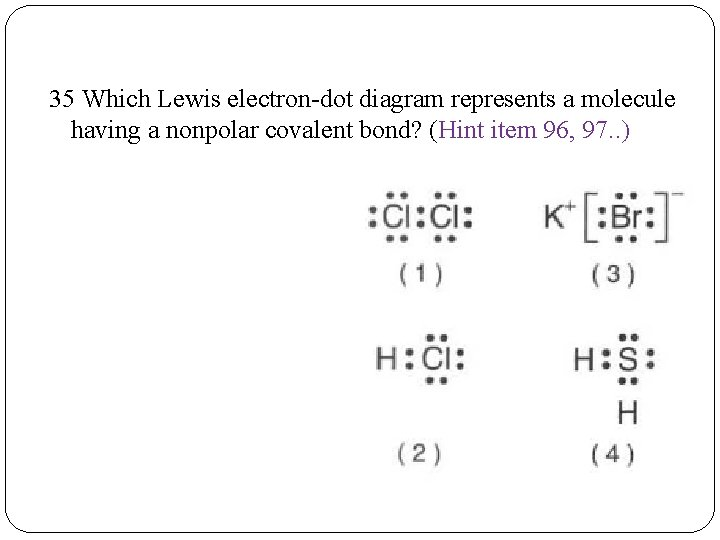35 Which Lewis electron-dot diagram represents a molecule having a nonpolar covalent bond? (Hint item 96, 97. . )35 Which Lewis electron-dot diagram represents a molecule having a nonpolar covalent bond? (Items 96, 97 of 200 ways. . ) Remember molecules have covalent bonds; Bond polarity increases as the difference in electronegativity between the bonded atoms increases; Bonds between identical atoms will be nonpolar because the difference in electronegativity is zero The best answer here would be Cl 2 (E. D. = 3. 2 -3. 2=0)• Q 36: Metric prefixes and Scientific notation ( Item 23 and reference table C) 36 Which quantity is equal to 50 kilojoules? (I ) 0. 05 J (3) 5 x 103 J (2) 500 J (4) 5 x 104 J 37 Which compound is formed from its elements by an exothermic reaction at 298 K and 101. 3 k. Pa? (HINT: This is a table I question) (1) C 2 H 4(g) (2) HI(g) (3) H 2 O(g) (4) NO 2(g) 38 At which temperature is the vapor pressure of ethanol equal to 80. k. Pa? (You Must Use Vapor pressure Curves Table – Table H) (1) 48°C (3) 80. °C (2) 73°C (4) 101 °C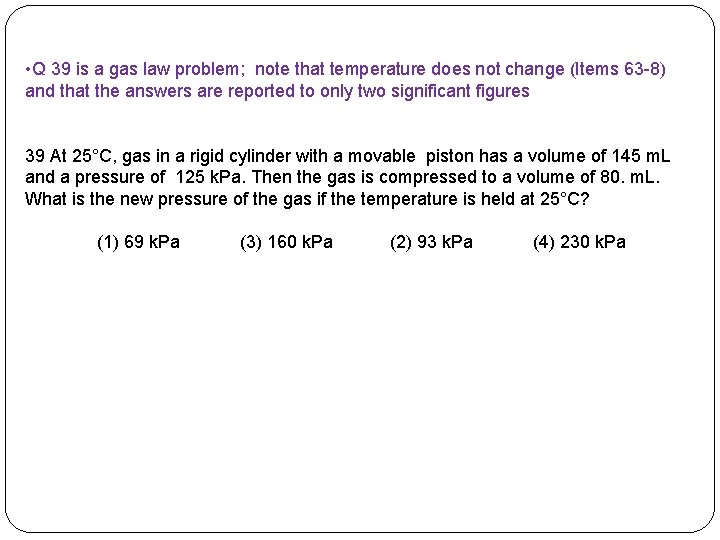• Q 39 is a gas law problem; note that temperature does not change (Items 63 -8) and that the answers are reported to only two significant figures 39 At 25°C, gas in a rigid cylinder with a movable piston has a volume of 145 m. L and a pressure of 125 k. Pa. Then the gas is compressed to a volume of 80. m. L. What is the new pressure of the gas if the temperature is held at 25°C? (1) 69 k. Pa (3) 160 k. Pa (2) 93 k. Pa (4) 230 k. Pa• Q 39 is a gas law problem; note that temperature does not change (Items 63 -8) and that the answers are reported to only two significant figures 39 At 25°C, gas in a rigid cylinder with a movable piston has a volume of 145 m. L and a pressure of 125 k. Pa. Then the gas is compressed to a volume of 80. m. L. What is the new pressure of the gas if the temperature is held at 25°C? (1) 69 k. Pa (3) 160 k. Pa (2) 93 k. Pa (4) 230 k. Pa Start with Combined gas law from Table T. Remember Temperature in Kelvin for gas law problems. For this problem, Combined gas law can be simplified because Temperature is constant so Use P 1 V 1 = P 2 V 2 to Solve. Equation can be rearranged first to P 2 = P 1 V 1 V 2 in order to solve for P 2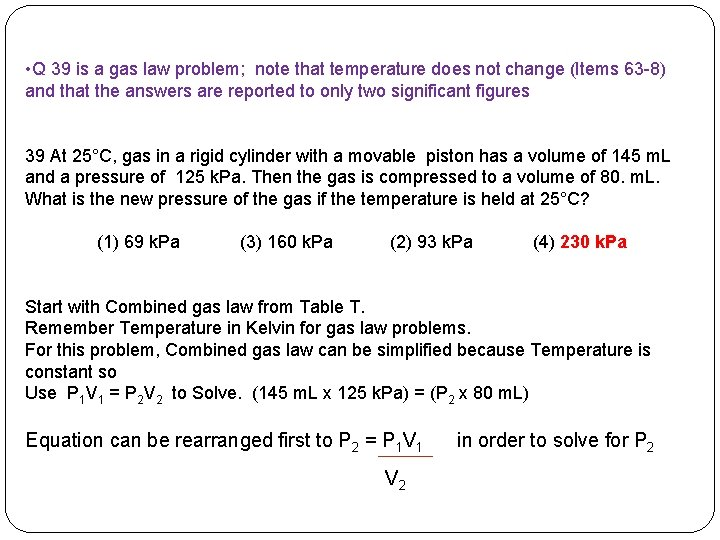• Q 39 is a gas law problem; note that temperature does not change (Items 63 -8) and that the answers are reported to only two significant figures 39 At 25°C, gas in a rigid cylinder with a movable piston has a volume of 145 m. L and a pressure of 125 k. Pa. Then the gas is compressed to a volume of 80. m. L. What is the new pressure of the gas if the temperature is held at 25°C? (1) 69 k. Pa (3) 160 k. Pa (2) 93 k. Pa (4) 230 k. Pa Start with Combined gas law from Table T. Remember Temperature in Kelvin for gas law problems. For this problem, Combined gas law can be simplified because Temperature is constant so Use P 1 V 1 = P 2 V 2 to Solve. (145 m. L x 125 k. Pa) = (P 2 x 80 m. L) Equation can be rearranged first to P 2 = P 1 V 1 V 2 in order to solve for P 2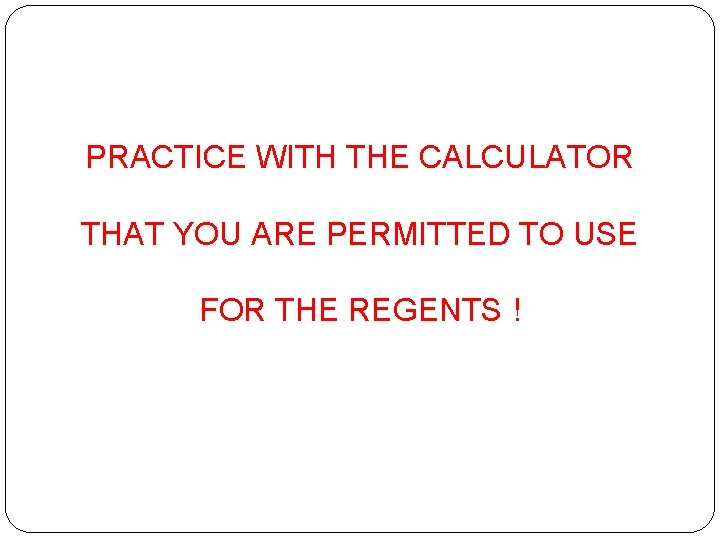PRACTICE WITH THE CALCULATOR THAT YOU ARE PERMITTED TO USE FOR THE REGENTS !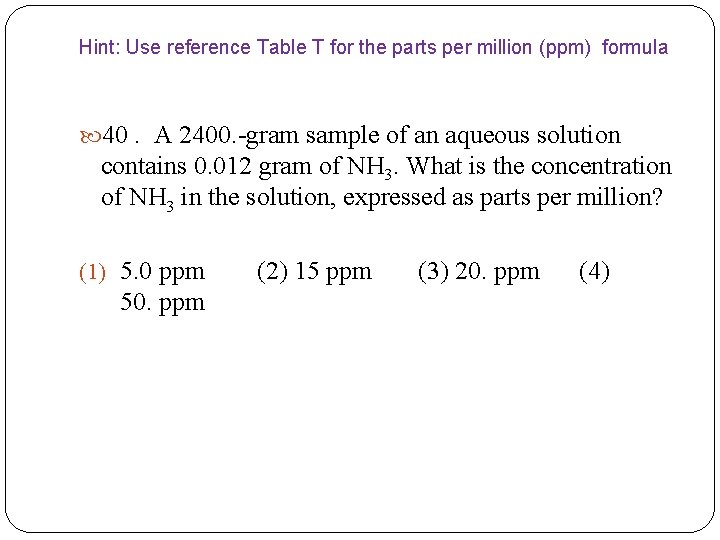Hint: Use reference Table T for the parts per million (ppm) formula 40. A 2400. -gram sample of an aqueous solution contains 0. 012 gram of NH 3. What is the concentration of NH 3 in the solution, expressed as parts per million? (1) 5. 0 ppm (2) 15 ppm (3) 20. ppm (4) 50. ppm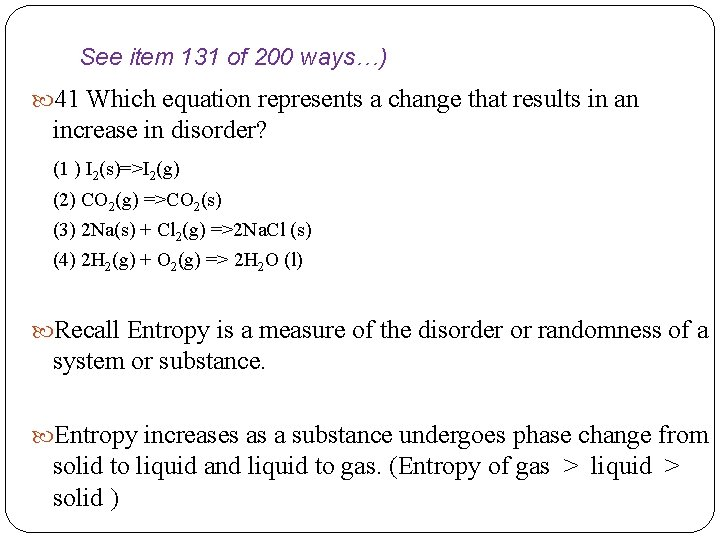See item 131 of 200 ways…) 41 Which equation represents a change that results in an increase in disorder? (1 ) I 2(s)=>I 2(g) (2) CO 2(g) =>CO 2(s) (3) 2 Na(s) + Cl 2(g) =>2 Na. Cl (s) (4) 2 H 2(g) + O 2(g) => 2 H 2 O (l) Recall Entropy is a measure of the disorder or randomness of a system or substance. Entropy increases as a substance undergoes phase change from solid to liquid and liquid to gas. (Entropy of gas > liquid > solid )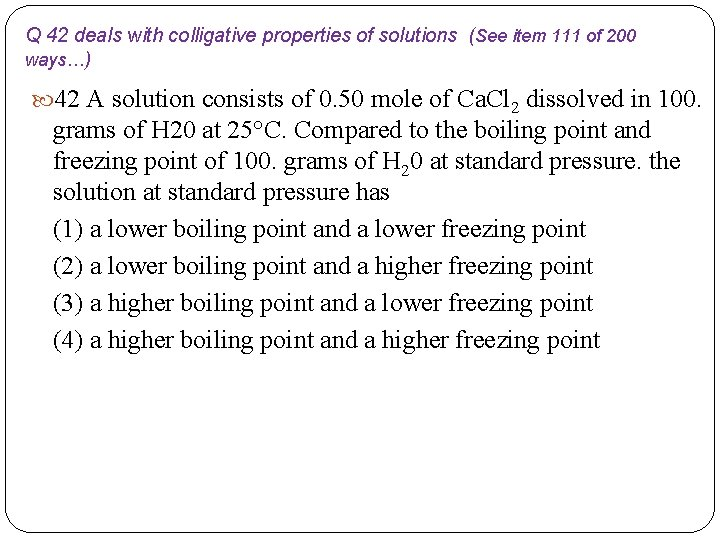Q 42 deals with colligative properties of solutions (See item 111 of 200 ways…) 42 A solution consists of 0. 50 mole of Ca. Cl 2 dissolved in 100. grams of H 20 at 25°C. Compared to the boiling point and freezing point of 100. grams of H 20 at standard pressure. the solution at standard pressure has (1) a lower boiling point and a lower freezing point (2) a lower boiling point and a higher freezing point (3) a higher boiling point and a lower freezing point (4) a higher boiling point and a higher freezing point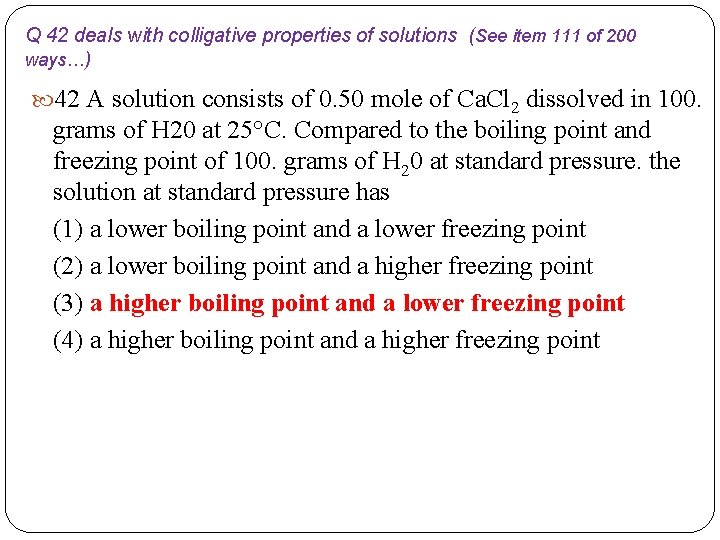Q 42 deals with colligative properties of solutions (See item 111 of 200 ways…) 42 A solution consists of 0. 50 mole of Ca. Cl 2 dissolved in 100. grams of H 20 at 25°C. Compared to the boiling point and freezing point of 100. grams of H 20 at standard pressure. the solution at standard pressure has (1) a lower boiling point and a lower freezing point (2) a lower boiling point and a higher freezing point (3) a higher boiling point and a lower freezing point (4) a higher boiling point and a higher freezing point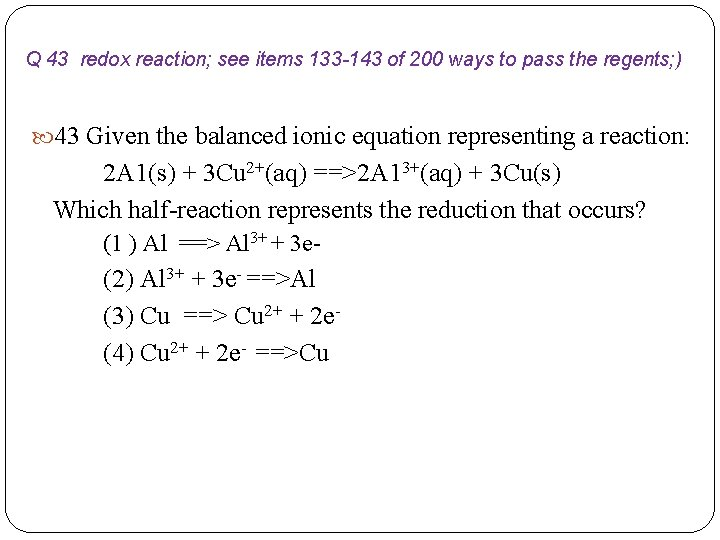Q 43 redox reaction; see items 133 -143 of 200 ways to pass the regents; ) 43 Given the balanced ionic equation representing a reaction: 2 A 1(s) + 3 Cu 2+(aq) ==>2 A 13+(aq) + 3 Cu(s) Which half-reaction represents the reduction that occurs? (1 ) Al ==> Al 3+ + 3 e- (2) Al 3+ + 3 e- ==>Al (3) Cu ==> Cu 2+ + 2 e(4) Cu 2+ + 2 e- ==>Cu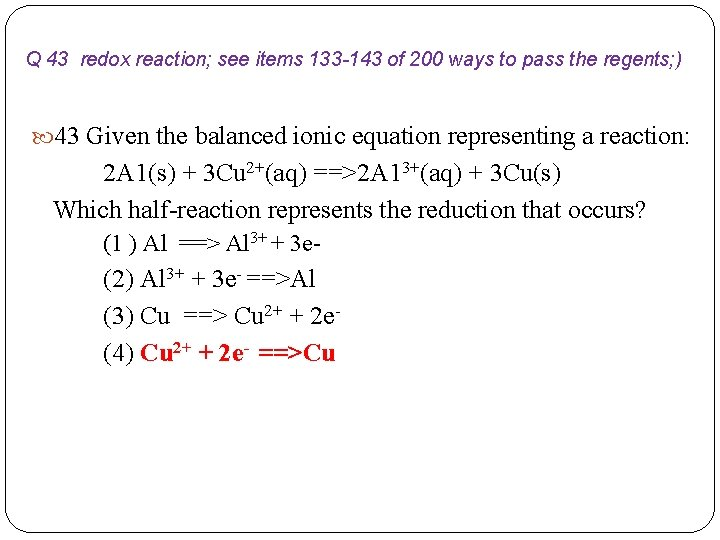Q 43 redox reaction; see items 133 -143 of 200 ways to pass the regents; ) 43 Given the balanced ionic equation representing a reaction: 2 A 1(s) + 3 Cu 2+(aq) ==>2 A 13+(aq) + 3 Cu(s) Which half-reaction represents the reduction that occurs? (1 ) Al ==> Al 3+ + 3 e- (2) Al 3+ + 3 e- ==>Al (3) Cu ==> Cu 2+ + 2 e(4) Cu 2+ + 2 e- ==>Cu44 Given the equation and potential energy diagram representing a reaction: If each interval on the axis labeled "Potential Energy (k. J/mol)" represents 10. k. J/mol, what is the heat of reaction? (1) +60. k. J/mol (2) +20. k. J/mol (3) +30. k. J/mol (4) +40. k. J/mol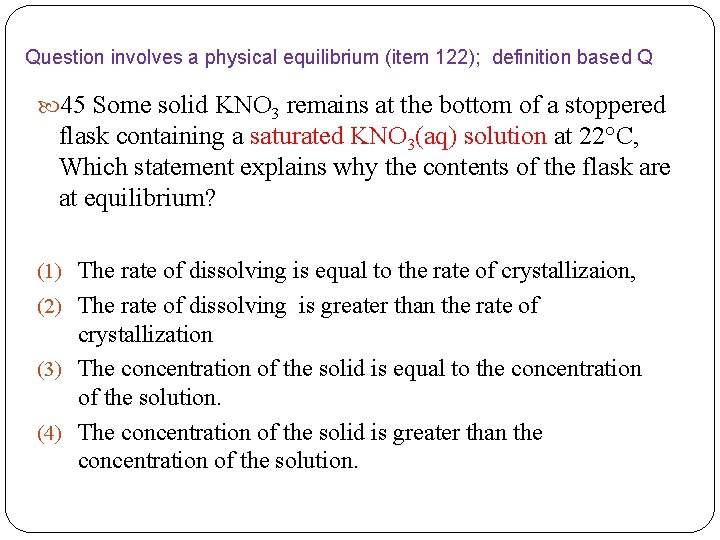Question involves a physical equilibrium (item 122); definition based Q 45 Some solid KNO 3 remains at the bottom of a stoppered flask containing a saturated KNO 3(aq) solution at 22°C, Which statement explains why the contents of the flask are at equilibrium? (1) The rate of dissolving is equal to the rate of crystallizaion, (2) The rate of dissolving is greater than the rate of crystallization (3) The concentration of the solid is equal to the concentration of the solution. (4) The concentration of the solid is greater than the concentration of the solution.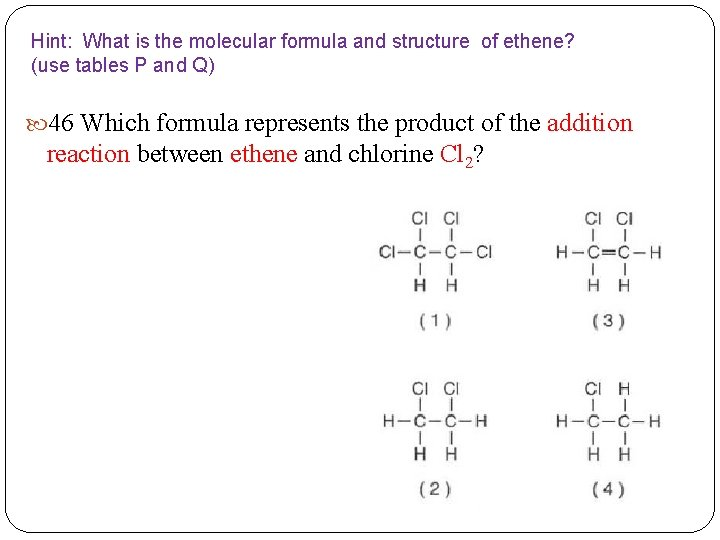Hint: What is the molecular formula and structure of ethene? (use tables P and Q) 46 Which formula represents the product of the addition reaction between ethene and chlorine Cl 2?Use table J; For each equation , on the reactants side, compare the position of the metallic element to the metal cation in the ionic compound. Look for the most reactive metal to be the species that is oxidized in the reaction; it should be the metal element on the left of the equation) 47 Based on Reference Table J, which two reactants react spontaneously? (I) Mg(s) + Zn. Cl 2 (aq) (2) Cu(s) + Fe. SO 4(aq) (3) Pb(s) + Zn. Cl 2(aq) (4) Co(s)+ Na. Cl(aq)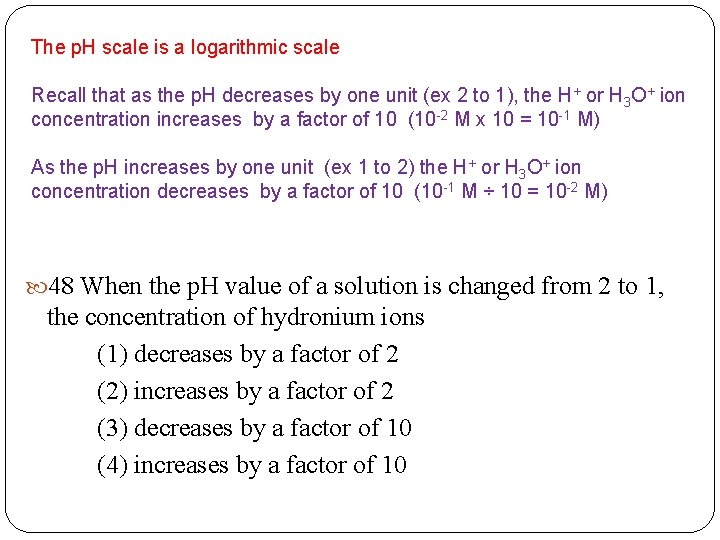The p. H scale is a logarithmic scale Recall that as the p. H decreases by one unit (ex 2 to 1), the H+ or H 3 O+ ion concentration increases by a factor of 10 (10 -2 M x 10 = 10 -1 M) As the p. H increases by one unit (ex 1 to 2) the H+ or H 3 O+ ion concentration decreases by a factor of 10 (10 -1 M ÷ 10 = 10 -2 M) 48 When the p. H value of a solution is changed from 2 to 1, the concentration of hydronium ions (1) decreases by a factor of 2 (2) increases by a factor of 2 (3) decreases by a factor of 10 (4) increases by a factor of 10Q involves nuclear reactions (Items 177 -189; see item 182 in particular) 49 Given the balanced equation representing a nuclear reaction: Which phrase identifies and describes this reaction? (1) fission, mass converted to energy (2) fission, energy converted to mass (3) fusion, mass converted to energy (4) fusion, energy converted to massQuestion involves Bronsted Lowry definition of acids and bases; items 153 of 200 ways…. ) 50 Given the equation representing a reversible reaction: NH 3(g) + H 2 O(l) <=> NH 4+(aq) + OH-(aq) According to one acid-base theory, the reactant that donates an H+ ion in the forward reaction is (1) NH 3(g) (2) H 2 O(l) (3) NH 4+(aq) (4) OH-(aq)